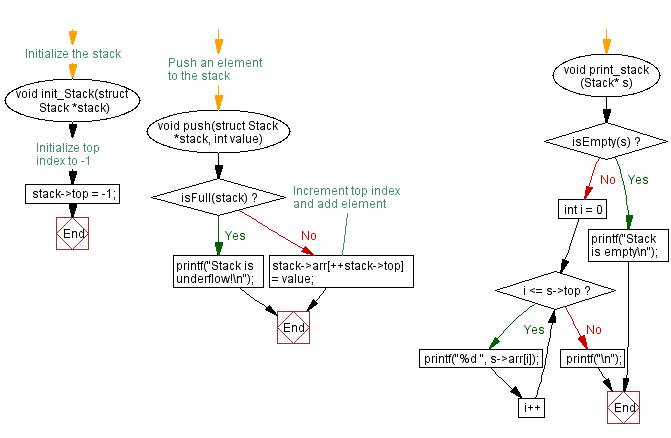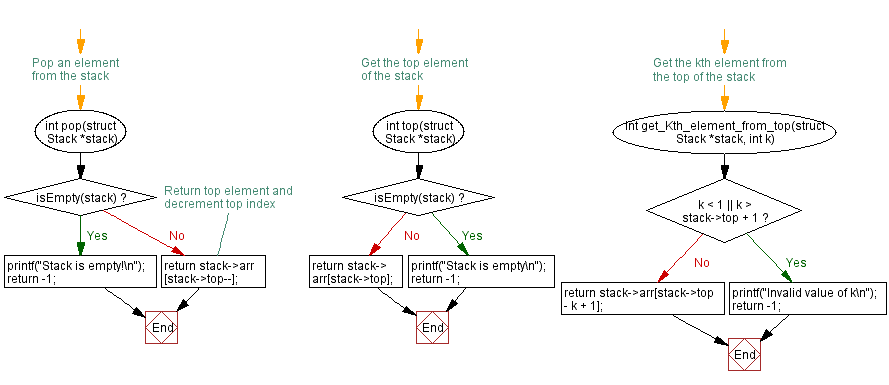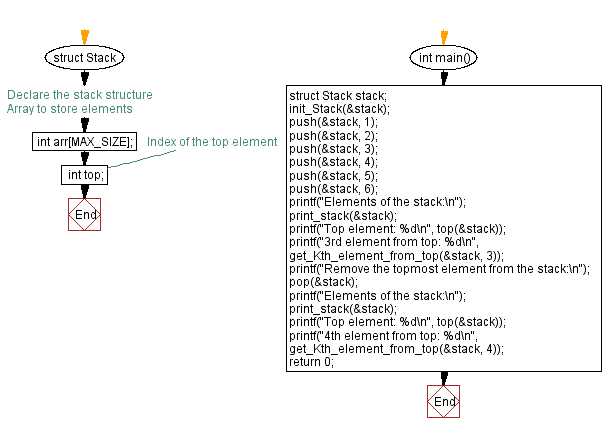﻿ C - Find the top and kth element of a stack

# C Exercises: Find the top and kth element of a stack

## C Stack: Exercise-16 with Solution

Write a C program to implement a stack and accept some numeric values. Find the top and kth element of the stack.

Sample Solution:

C Code:

``````#include <stdio.h>
#include <stdlib.h>

#define MAX_SIZE 100

// Declare the stack structure
struct Stack {
int arr[MAX_SIZE]; // Array to store elements
int top; // Index of the top element
};

// Initialize the stack
void init_Stack(struct Stack *stack) {
stack->top = -1; // Initialize top index to -1
}

// Check if stack is empty
int isEmpty(struct Stack *stack) {
return (stack->top == -1);
}

// Check if stack is full
int isFull(struct Stack *stack) {
return (stack->top == MAX_SIZE - 1);
}

// Push an element to the stack
void push(struct Stack *stack, int value) {
if (isFull(stack)) {
printf("Stack is underflow!\n");
return;
}
stack->arr[++stack->top] = value; // Increment top index and add element
}

// Pop an element from the stack
int pop(struct Stack *stack) {
if (isEmpty(stack)) {
printf("Stack is empty!\n");
return -1;
}
}

// Get the top element of the stack
int top(struct Stack *stack) {
if (isEmpty(stack)) {
printf("Stack is empty\n");
return -1;
}
return stack->arr[stack->top];
}

// Get the kth element from the top of the stack
int get_Kth_element_from_top(struct Stack *stack, int k) {
if (k < 1 || k > stack->top + 1) {
printf("Invalid value of k\n");
return -1;
}
return stack->arr[stack->top - k + 1];
}

void print_stack(Stack* s) {
if (isEmpty(s)) {
printf("Stack is empty\n");
return;
}
for (int i = 0; i <= s->top; i++) {
printf("%d ", s->arr[i]);
}
printf("\n");
}

int main() {
struct Stack stack;
init_Stack(&stack);
push(&stack, 1);
push(&stack, 2);
push(&stack, 3);
push(&stack, 4);
push(&stack, 5);
push(&stack, 6);
printf("Elements of the stack:\n");
print_stack(&stack);
printf("Top element: %d\n", top(&stack));
printf("3rd element from top: %d\n", get_Kth_element_from_top(&stack, 3));
printf("Remove the topmost element from the stack:\n");
pop(&stack);
printf("Elements of the stack:\n");
print_stack(&stack);
printf("Top element: %d\n", top(&stack));
printf("4th element from top: %d\n", get_Kth_element_from_top(&stack, 4));
return 0;
}
```
```

Sample Output:

```Elements of the stack:
1 2 3 4 5 6
Top element: 6
3rd element from top: 4
Remove the topmost element from the stack:
Elements of the stack:
1 2 3 4 5
Top element: 5
4th element from top: 2
```

Flowchart:C Programming Code Editor:

Have another way to solve this solution? Contribute your code (and comments) through Disqus.

What is the difficulty level of this exercise?

Test your Programming skills with w3resource's quiz.

﻿

## C Programming: Tips of the Day

C Programming - How do you pass a function as a parameter in C?

Declaration

A prototype for a function which takes a function parameter looks like the following:

```void func ( void (*f)(int) );
```

This states that the parameter f will be a pointer to a function which has a void return type and which takes a single int parameter. The following function (print) is an example of a function which could be passed to func as a parameter because it is the proper type:

```void print ( int x ) {
printf("%d\n", x);
}
```

Function Call

When calling a function with a function parameter, the value passed must be a pointer to a function. Use the function's name (without parentheses) for this:

```func(print);
```

would call func, passing the print function to it.

Function Body

As with any parameter, func can now use the parameter's name in the function body to access the value of the parameter. Let's say that func will apply the function it is passed to the numbers 0-4. Consider, first, what the loop would look like to call print directly:

```for ( int ctr = 0 ; ctr < 5 ; ctr++ ) {
print(ctr);
}
```

Since func's parameter declaration says that f is the name for a pointer to the desired function, we recall first that if f is a pointer then *f is the thing that f points to (i.e. the function print in this case). As a result, just replace every occurrence of print in the loop above with *f:

```void func ( void (*f)(int) ) {
for ( int ctr = 0 ; ctr < 5 ; ctr++ ) {
(*f)(ctr);
}
}
```

Ref : https://bit.ly/3skw9Um